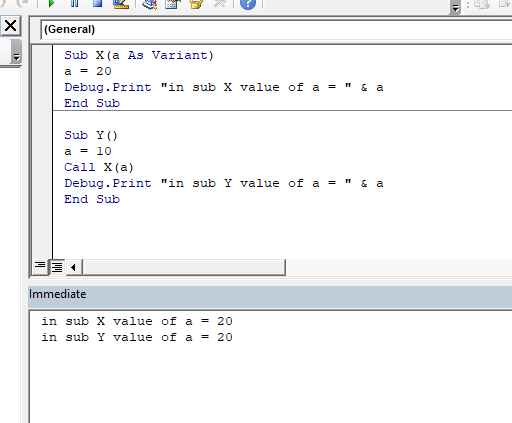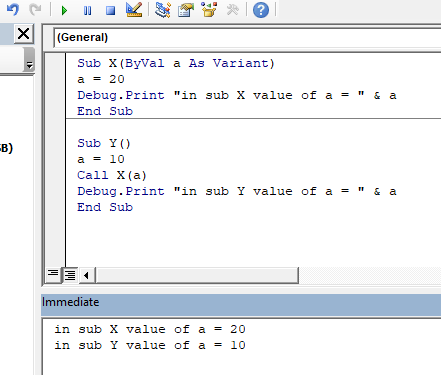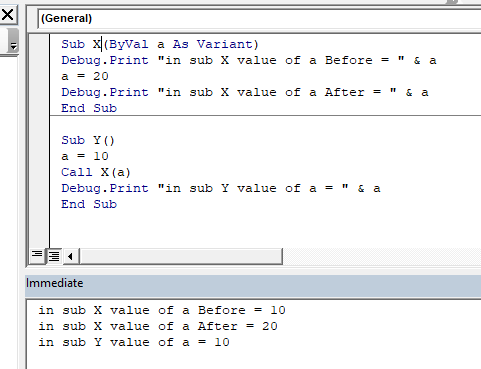# What is the difference Between ByRef and ByVal Arguments? | VBA Interview Question

This is one of most asked questions in VBA interviews. In this article we will learn what’s the difference between ByVal and ByRef arguments in excel VBA.

## Definitions:

ByRef argument: It is the literal short form of by reference. When an argument is passed as ByRef argument to a different sub or function, the reference of the actual variable is sent. Any changes made in to the copy of variable, will reflect in original argument.
We can say that, instead of value, the location of value is sent to function using ByRef to a function.
This is the default argument in VBA. We don’t need to write ByRef before argument.
Syntax:

Sub x(a as Variant)

‘Or

Sub x(ByRef a as Variant)

ByVal argument: It is a literal short form of by value. When an argument is passed as ByVal argument to a different sub or function, only the value of the argument is sent. The original argument is left intact. Any changes made in foreign function or sub will not reflect in the original argument.
To declare an argument as ByVal you need to use the ByVal keyword before the argument.
Syntax:
Sub x(ByVal a as Variant)
Now we know the definitions. Let’s see an example and get over with it.

## ByRef Example

Here’s a simple program.

```Sub X(ByRef a As Variant)
a = 20
Debug.Print "in sub X value of a = " & a
End Sub

Sub Y()
a = 10
Call X(a)
Debug.Print "in sub Y value of a = " & a
End Sub
```

So, here we have two subroutines. First sub is X that takes a variant argument as ByRef.
(You can omit the ByRef keyword. It’s the default.)
Next, it is setting the value of a = 20 end then prints the value of a.

Sub Y is the main subroutine that calls subroutine X. It sets the value of a=10 then calls subroutine X and passes a as an argument. Then it prints value of a in Y.

Now when you run Sub Y this is the output you get.
in sub X value of a = 20

in sub Y value of a = 20Conclusion:  The value of original a is changed by sub X and set to 20 for both subs.
you can see that when Sub Y runs, the initial value of a was 10. Y calls X(a). X sets value of a=20. It prints “in sub X value of a = 20”. Control goes back to y and prints in sub Y value of a = 20.
This is the effect of ByRef argument.

## ByVal Example:

This is a ByVal Example

```Sub X(ByVal a As Variant)
a = 20
Debug.Print "in sub X value of a = " & a
End Sub

Sub Y()
a = 10
Call X(a)
Debug.Print "in sub Y value of a = " & a
End Sub```

Both examples are same with the only difference of argument passing. Here in X, arguments are declared as ByVal. When you run Y subroutine this time the output is:

in sub X value of a = 20
in sub Y value of a = 10Conclusion:  The value of original a is NOT changed by sub X. It is 20 for X and 10 For Y.

When Y call X with a, it only sends the value of a not the address of a. Hence any change made in a is not reflected in the original variable.

For test, if print value of a in X before setting it to 20, it will print 10. Because the 10 is passed to a in X using byVal. Using ByRef you sent a of Y to a of X.It is an easy question, but many of us confuse this, in vba interview question. The reason is that we don’t get to use it much. Most of the time we copy the value in different variable

So yeah guys, this is the difference bw ByRef and ByVal arguments in excel VBA. Let me know, if you have any doubt regarding this topic or any other VBA or Excel topic. The comments section is all yours.

Add And Save New Workbook Using VBA In Microsoft Excel 2016

Display A Message On The Excel VBA Status Bar

Turn Off Warning Messages Using VBA In Microsoft Excel 2016

Popular Articles:

The VLOOKUP Function in Excel

COUNTIF in Excel 2016

How to Use SUMIF Function in Excel

Terms and Conditions of use

The applications/code on this site are distributed as is and without warranties or liability. In no event shall the owner of the copyrights, or the authors of the applications/code be liable for any loss of profit, any problems or any damage resulting from the use or evaluation of the applications/code.